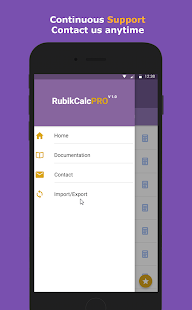RubikCalc is a unique, fully programmable calculator that allows you to add all your most used functions at the swipe of a screen, having a very user friendly interface so just about anyone can program this calculator to be a physical, scientific or mathematical in one single custom formula calculator.

You can use your custom formula converted into calculator in no time without any programming skills or experience, just math.

Features:
2- See the results in real-time while you type.
3- See the steps of the solution
4- Save your calculators to use them later
5- Supports Trigenometric functions (Sin,Cos,Tan … )
6- Supports Logarithmic functions

Use the following formula and see the results immediately in real-time debugging.

Example1 (Velocity Calculator)
Distance[m] = x
Time[s] = t
Velocity[m/s] = x/t

Example2 (Desnity Calculator):
Mass[kg]=m
Volume[m3] =v
Density[kg/m3] =m/v

Example3 (Volume Calculator):
Length=x
Width=y
Height=z
Volume=xyz
BaseArea=x*y

Example4 (Trigenometric Calculator):
Length=x
Width=y
Height=z
Volume=xyz
BaseArea=x*y

# FAQs:

• What is the “programmable custom formula Calculator”?
• It’s an app that allows you to write your custom formula and convert it to custom calculator easily.

• How RubikCalc is different from any other programmable calculator?

• RubikCalc is totally different from other programmable calculator so that it’s not a static app, it’s totally dynamic, you can create your own customized formula calculators and save it and use it later.

• Can I use variables names like this p1,a3?

• No, you cannot mix numbers with letters in variable names, just use single symbols a,b,c,x,y,

• How does the app distinguishes between inputs and outputs?

• Outputs contains formula like this: Velocity=x/t,Square=x^2
While Inputs are just definitions like this: Distance=x, Time=t, Radius=r

• How can i specify units?

• Put the units between brackets after the name like this, Distance[m]=x

• How can i use the logarithmic function?

• Just write log(3),log(4).

• Can i use PI ?

• Yes, just write PI, all capital.

What’s New:
BUG-FIX
UI ENHANCE
INTERNET PERMISSION TO SEND SURVEY

Screenshots

•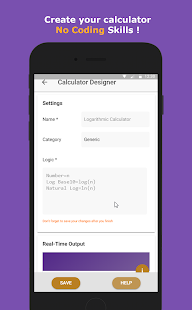•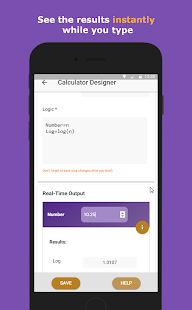•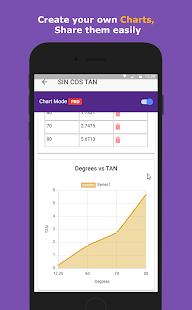•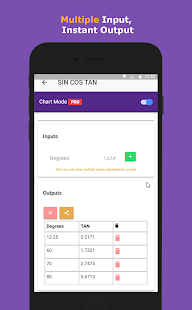•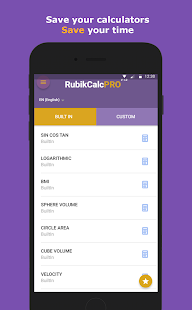••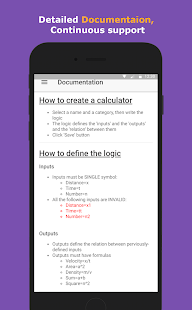•